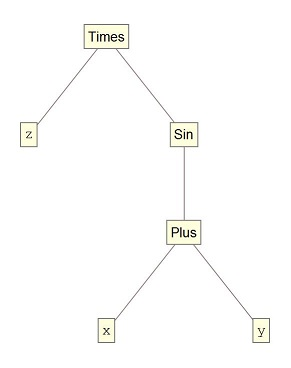# Neural Networks and QFT

\newcommand{\set}{\{#1\}} \newcommand{\pdv}{\frac{\partial #1}{\partial #2}} \newcommand{\myalign}{\begin{aligned}#1\end{aligned}} \newcommand{\myarray}{\begin{array}{#1}#2\end{array}} \renewcommand{\vev}{\langle #1\rangle} \newcommand{\p}{\partial} \newcommand{\n}{\nabla} \renewcommand{\inf}{\infty} \newcommand{\Diff}{\operatorname{Diff}} \newcommand{\Aut}{\operatorname{Aut}} \newcommand{\End}{\operatorname{End}} \newcommand{\Ind}{\operatorname{Ind}} \newcommand{\sign}{\operatorname{sign}} \renewcommand{\hbar}{\bar{h}} \newcommand{\wbar}{\bar{w}} \newcommand{\zbar}{\bar{z}} \renewcommand{\a}{\alpha} \renewcommand{\b}{\beta} \newcommand{\g}{\gamma} \newcommand{\G}{\Gamma} \renewcommand{\d}{\delta} \newcommand{\D}{\Delta} \newcommand{\e}{\epsilon} \newcommand{\ve}{\varepsilon} \renewcommand{\k}{\kappa} \renewcommand{\l}{\lambda} \renewcommand{\L}{\Lambda} \newcommand{\s}{\sigma} \renewcommand{\S}{\Sigma} \renewcommand{\r}{\rho} \renewcommand{\o}{\omega} \renewcommand{\O}{\Omega} \renewcommand{\th}{\theta} \newcommand{\Th}{\Theta} \renewcommand{\t}{\tau} \newcommand{\z}{\zeta} \newcommand{\mbf}{\mathbf{#1}} \newcommand{\mb}{\mathbb{#1}} \newcommand{\mc}{\mathcal{#1}} \newcommand{\mf}{\mathfrak{#1}} \newcommand{\R}{\mathbb{R}} \newcommand{\N}{\mathbb{N}} \newcommand{\Z}{\mathbb{Z}} \newcommand{\C}{\mathbb{C}} \newcommand{\V}{\mathbb{V}} \newcommand{\W}{\mathbb{W}} \newcommand{\cP}{\mathcal{P}} \newcommand{\cO}{\mathcal{O}} \newcommand{\cA}{\mathcal{A}} \newcommand{\cB}{\mathcal{B}} \newcommand{\cC}{\mathcal{C}} \newcommand{\cD}{\mathcal{D}} \newcommand{\cH}{\mathcal{H}} \newcommand{\cL}{\mathcal{L}} \newcommand{\glie}{\mathfrak{g}} \newcommand{\gllie}{\mathfrak{gl}} \newcommand{\sllie}{\mathfrak{sl}} \newcommand{\olie}{\mathfrak{o}} \newcommand{\solie}{\mathfrak{so}} \newcommand{\isolie}{\mathfrak{iso}} \newcommand{\splie}{\mathfrak{sp}} \newcommand{\nlie}{\mathfrak{n}} \newcommand{\plie}{\mathfrak{p}} \newcommand{\blie}{\mathfrak{b}} \newcommand{\hlie}{\mathfrak{h}} \newcommand{\klie}{\mathfrak{k}}

## Reiko1/21/2021, 3:51:19 PM

### 比喻

• 抽象或者具体的对象，不依赖于人类
• 特定人类群体对于该对象的认识
• “我”对于该对象的认识，即具体的神经细胞之间的连接和上面传导的相应的信号

Reiko1/21/2021, 3:55:19 PM

1111111111

Reiko1/21/2021, 3:58:52 PM

# QFT的一般意味

• locality,
• unitarity, positivity and causality,
• base manifold vs. target manifold,
• lattice vs. continuum,
• global symmetry and gauge structure, 等等。

Reiko1/21/2021, 4:06:02 PM

# Path integral as formal measure

## 为什么收敛性通常（不）是一个问题

1. 因为遇到的收敛性问题一般最终归约到了到$\mathbb{R}$的赋值，而之所以需要在$\mathbb{R}$上赋值，是因为现实世界在我们了解的尺度内很好的被$\mathbb{R}$上的数学/物理所描述。而EFT中的微扰计算，一般来说只关心到$\mathbb{R}[[h]]$的赋值，即计算结果是$h$的形式幂级数。

2. 另一个例子是关于Grassmann数的Berezin积分，目前为止这种积分还未能被理解成通常意义的测度，我们实际需要的是它bookkeeping了超空间的信息，以及$\int d\th \th$读出一个实数。尽管任意一个supermanifold都(non-canonically)同胚于一个exterior bundle。

3. 最后一个例子是在进行正规化的时候，我们一般在tempered distribution空间上进行计算，这上面的积分也已经失去了测度的直观意义。最简单的例子是$\frac{1}{x^2}$作为分布满足，

$\int_{\mathbb{R}}dx\,\frac{1}{x^2}=0$

我们实际需要的是分布在Fourier变换下的良好行为。Reiko1/21/2021, 6:00:16 PM

# Gaussian分布/平均场论/GFT

2-pt function/2-moment/Propagator/Green function/Kernel function/...

Reiko1/21/2021, 6:03:16 PM

# Elsewhere

11111111111

## Reiko replied to Knapp's book on semisimple groups

3 Smooth Vectors and the Universal Enveloping Algebra 定理: 对于李群G和实李代数glie，有U(glie^{C})和G上左不变微分算子D(G)的自然同构。 讨论紧李群的有限维表示时直接求（李）导数就可以给出对应李代数的表示。对于无穷维表示首先就遇到无界算子定义域的问题，例如考虑幺正表示的时候，回忆Stone定理，单参数幺正子群e^{t A}和反自伴算子A一一对应，考虑A的多项式时需要进一步缩小定义域。为此对于表示H定义光滑矢量vinH要满足， [gto r(g)v] 对于gin G是光滑的。光滑矢量空间记为C^{infty}(r)。 由此可定义r诱导的U(glie^{C})的表示， [r(X)v=lim_{tto 0}frac{r(e^{t X})v-v}{t}] 为了看到C^{infty}(r)在H中是稠密的，我们可以考虑Garding子空间，定义为r(f)v张成的空间。

## Reiko replied to Knapp's book on semisimple groups

2 Reps of Small Groups 我们用物理约定，和Knapp的符号差一点，

%%[begin{pmatrix}a&bc&dend{pmatrix}]%% begin{align} z'&=r(z)=frac{az+b}{cz+d}z&=-frac{dz-b}{cz-a} pdv{z'}{z}&=(cz+d)^{-2} D&=h+hbar=1+is D^S &=2-D=1-is J&=|h-hbar|infrac{1}{2}Z J^S&=-J hat{f}(p)&=int f(x) e^{-ipx} end{align} S for shadow, D for dimension and J for spin. Unitary Irreps of SL(2,C) （幺正）主列表示r_{D,J}作用在H=L^2(C)上，

begin{align} rbegin{pmatrix}a&bc&dend{pmatrix}f(z,zbar)&=(pdv{z'}{z})^h (pdv{zbar'}{zbar})^{hbar}f(z',zbar') &=(cz+d)^{-2h}(bar{c}zbar+bar{d})^{-2hbar}f(z',zbar') &=|cz+d|^{-2-2is}arg^{2J}(cz+d)f(z',zbar') end{align} J的取值保证单值性，遵循物理中primary算子的变换关系，但需要把量纲延拓到主列上。在单位元附近的连续性可用控制收敛定理看出。这里也可以用共形紧化提升到线性作用。 对于SO(3,1)cong PSL(2,C)=SL(2,C)backslash set{pm 1}，单值性限制自旋为整数。 主列表示的幺正性：定义内积为， [(f,g)=int f g^{*}] D的实部贡献和Jacobian相消，因此是不变内积。 讨论： 补充列表示的内积需要另行定义。SL(2,C)没有离散列； 物理中Hilbert空间的幺正性（以及给出的幺正下界）对应Lorentzian共形群的幺正性，或者说原点表示和无穷远表示配对的正定性，在这种意义下主列是非幺正的（出现在celestial CFT中）。 主列表示的不可约性：由平移，旋转和伸缩生成的上三角子群已经可以说明不可约性（这点来自于诱导表示）。下面计算和上三角子群交换的算子A必须为常数算子。 和平移交换(Af)(z+a)=A(f(z+a))在Fourier空间为， [hat{Af}] 略去 Unitary Irreps of SL(2,R) 限定在z=zbar上。 离散列

## Colliot replied to 文章名字至少要十个字

stacks project 好像用的是 XyJax（一个 xy-pic 的实现） 来做交换图。